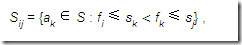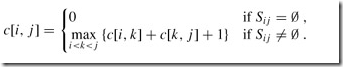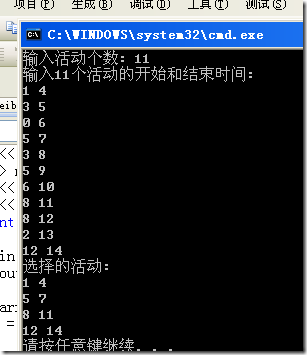# 《算法导论》学习总结 — 22.第16章 贪心算法(2) 案例分析之活动选择问题

08 Jun 2011Tanky Woo 标签: Tanky Woo贪心算法算法导论

(其实贪心这块和DP一样，很难说清楚，看多了，做多了，你就知道为何这个问题可以用DP，这个问题可以用贪心。。。)

``````#include
#include
using namespace std;

typedef struct Act{
int s;   // start time
int f;   // final time
}Act;

Act arr;
Act temp;
int n;

bool cmp(Act a, Act b)
{
return a.f < b.f;
}

int main()
{
//freopen("input.txt", "r", stdin);
cout << "输入活动个数: ";
cin >> n;
cout << "输入" << n << "个活动的开始和结束时间:" << endl;
for(int i=0; i> arr[i].s >> arr[i].f;
sort(arr, arr+n, cmp);
int k = 0;

temp[k] = arr;
for(int i=1; i= temp[k].f)
temp[++k] = arr[i];

cout << "选择的活动: " << endl;
for(int i=0; i<=k; ++i)
cout << temp[i].s << " " << temp[i].f << endl;
return 0;
}
``````